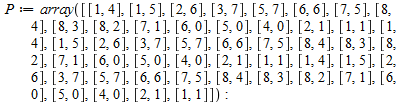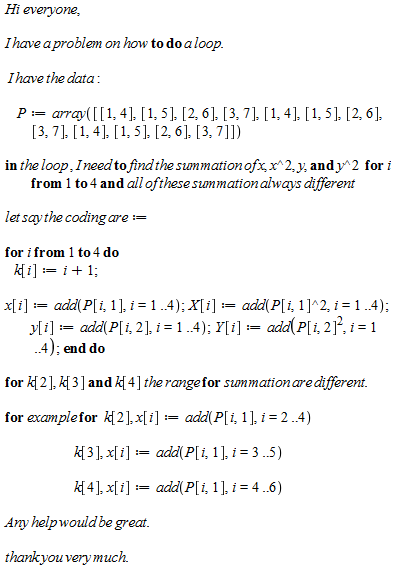## how do I solve a distinct-degree decomposition pro...

Trace  on computing the distinct-degree decomposition of the squarefree polynomial
f=x^17+2x^15+4x^13+x^12+2x^11+2x^10+3x^9+4x^4+3x^3+2x^2+4x belong to F_5[x].tell from the output only how many irreducible factors of degree i the polynomial f has, for all i.

## Simplifying expression with exponentials...

I am new to Maple and I have difficulties simplifying expressions like the following: (exp(a+b)+exp(a+c))*exp(-a).

I would expect to see exp(b)+exp(c) but nothing happens when I use the simplify() function.

I googled but didn't find a solution.

## to solve a partial differential equation with mapl...

hey i am new here and i have a question,

i have an partial differential equation diff(u(t,x),t\$2)=diff(u(t,x),x\$2) with the intial value problem u(0,x)=f(x)=1/(1+x^2) and diff(u(0,x),t)=0

and now my question i have already programm it:

> with(inttrans);

with(DEtools);

with(plots);

> with(PDETools);

> k := diff(u(t, x), `\$`(t, 2)) = diff(u(t, x), `\$`(x, 2));
bc := u(0, x) = 1/(1+x^2);
v := diff(u(0, x), t) = 0;
d / d \ d / d \
--- |--- u(t, x)| = --- |--- u(t, x)|
dt \ dt / dx \ dx /
1
u(0, x) = ------
2
1 + x
0 = 0

> pdsolve(k, u(t, x));
print(`output redirected...`);
u(t, x) = _F1(x + t) + _F2(x - t)

> c := pdsolve({bc, k, v}, u(t, x));
print(`output redirected...`); # input placeholder

and now question at the last there is nothing does it means that maple can´t solve it with the intial value problem and how can solve it with Fourier-Transformation to x???

can anyone help me please and sorry my englisch is not so good ;)

## how to create a matrix ...

Hi!

I still have a problem and im looking forward to any suggestions

this is the previous code that i have wrote

P := array([[8, 4], [8, 3], [8, 2], [7, 1], [6, 0], [5, 0], [4, 0], [2, 1], [1, 1], [1, 4]]);

> for j from 2 to 5 do k[j] := j+1;

x[j] := add(P[j, 1], j = j-1 .. j+2);

X[j] := add(P[j, 1]^2, j = j-1 .. j+2);

y[j] := add(P[j, 2], j = j-1 .. j+2);

Y[j] := add(P[j, 2]^2, j = j-1 .. j+2);

xy[j] := add(P[j, 1]*P[j, 2], j = j-1 .. j+2);

cx[j] := evalf(x[j]/k[j]);

cy[j] := evalf(y[j]/k[j]);

c11[j] := evalf(X[j]/k[j]-cx[j]^2);

c22[j] := evalf(Y[j]/k[j]-cy[j]^2);

c12[j] := evalf(xy[j]/k[j]-cx[j]*cy[j]);

C[j] := evalf(Matrix(2, 2, [[c11[j], c12[j]], [c12[j], c22[j]]]));

E[j] := simplify(fnormal(LinearAlgebra[Eigenvalues](C[j])));

if E[j] > E[j] then a[j] := E[j]/(E[j]+E[j]) else b[j] := E[j]/(E[j]+E[j])

end if;

a[j];b[j]

end do;

now, my question is how to put the output from the above looping in a  matrix form.

in my matrix, i need to call a[j],b[j], E[j], E[j] and the coordinate points. so my matrix dimension 4 x 5

I guess its a simple task but i tried hard and didnt get it worked.

thank you.

## what's wrong with this code?...

hi, I have tried to run this program unfortunately the program give some errors.

anneal < -function(fun, N, rho, t, theta)

{y=NULL

theta.old=theta repea {

s=0  for k from 1 to N

{

f.old=fun(theta.old)

theta.new=runif(1,-1,1)+theta.old

f.new=fun(theta.new)

if(f.new<f.old){

theta.star=theta.new

s=s+1

}

else

{

u=runif(1)

b=(u<=exp(-(f.new-f.old)/(t)))

theta.star=theta.new*b+theta.old*(1-b)

s=s+b

}

theta.old=theta.star

y=c(y,theta.star)

}

write(y,file="anneal.result",ncol=1,append=T)

y=NULL

if(s==0)break

t=rho*t

if(t<0.1)break  }

return(y)

}

cauchy.1=function(alpha){x=c(-4.20,-2.85,-2.30,-1.02,0.70,0.98,2.72,3.50)  return(sum(log(0.01+(x-alpha)^(2))))}

x=seq(-6,6,,300)

y=sapply(as.list(x),cauchy.1)

plot(x, y, type = "1", ylab = "cauchy.1(beta=.1,alpha)", xlab = "alpha")

anneal.result=anneal(cauchy.1,300,.95,10,4)

plot(x, y, type = "1", ylab = "cauchy.1(beta=.1,alpha)", xlab = "alpha")

anneal.result=anneal(cauchy.1,300,.95,10,4)

x = scan("anneal.result")

plot(1:length(x),x,type="1"

can anyone help me on this? can i do something about it

Many thanks.

## Coupled pde & ode solution in maple...

Hi everyone,

I have been  trying to solve a coupled system of 2 differencial equations, 1 PDEs with 1 ODE.The code is below.

> restart;

> with(linalg):with(plots):
> PDE:=[diff(x(z,t),t)=(a/Pe)*diff(x(z,t),z\$2)-a*diff(x(z,t),z)-(1-epsilon)/epsilon*3*Bi*(x(z,t)-1)/(1-Bi*(1-1/ksi(z,t)))]:
> a=2:Pe=3:Bi=5:epsilon=0.85:

> ODE := [diff(ksi(z,t),t) = (b*Bi*x(z,t)-1)/ksi(z,t)^2/(1-Bi*(1-1/ksi(z,t)))]:
> IC1:=c(z,0)=0:
> IC2:=ksi(z,0)=1:
> bc2:=diff(x(z,t),z):
> bc1:=x(z,t)-1/pe*diff(x(z,t),z):
> N:=10:
> L:=1:
> dyduf:=1/2*(-x(t)-3*x(t)+4*x(t))/h:
> dydub:=1/2*(-x[N-1](t)+3*x[N+1](t)+4*x[N](t))/h:
> dydu:=1/2/h*(x[m+1](t)-x[m-1](t)):
> d2ydu2:=1/h^2*(x[m-1](t)-2*x[m](t)+x[m+1](t)):
> bc1:=subs(diff(x(z,t),z)=dyduf,x(z,t)=x(t),z=1,bc1):
> bc2:=subs(x(z,t)-1/pe*diff(x(z,t),z)=dydub,x(z,t)=x[N+1](t),t=0,bc2):
> eq:=bc1:
> eq[N+1]:=bc2:
> eq[m]:=subs(diff(x(z,t),z\$2)=d2ydu2,diff(x(z,t),z)=dydu,diff(x(z,t),t)=dydu,x(z,t)=x[m](t),z=m*h,PDE):
> for i from 1 to N do eq[i]:=subs (m=i,eq[m]);od:
> x(t):=(solve(eq,x(t)));

> x[N+1](t):=solve(eq[N+1],x[N+1](t));

> for i from 1 to N do eq[i]:=eval(eq[i]);od:
> eqs:=[seq((eq[i]),i=1..N)]:
> Y:=[seq(x[i](t),i=1..N)];

> A:=genmatrix(eqs,Y,'B1'):
Error, (in linalg:-genmatrix) equations are not linear

> evalm(B1);

[B1, B1, B1, B1, B1, B1, B1, B1, B1,

B1]

> B:=matrix(N,1):for i to N do B[i,1]:=B1[i]:od:evalm(B);

> h:=eval(L/(N+1));

> A:=map(eval,A);

> if N > 10 then A:=map(evalf,A);end:
> evalm(A);

> mat:=exponential(A,t);
Error, (in linalg:-matfunc) input must be a matrix

> mat:=map(evalf,mat):
> Y0:=matrix(N,1):for i from 1 to N do
> Y0[i,1]:=evalf(subs(x=i*h,rhs(IC)));od:evalm(Y0);
Error, invalid input: rhs received IC, which is not valid for its 1st argument, expr

> s1:=evalm(Y0+inverse(A)&*b):
Error, (in linalg:-inverse) expecting a matrix

> Y:=evalm(mat&*s1-inverse(A)&*b):
Error, (in linalg:-inverse) expecting a matrix

> Y:=map(simplify,Y):
> Digits:=5;

Digits := 5

> for i from 1 to N do x[i](t):=evalf((Y[i,1]));od:
Error, invalid subscript selector

> for i from 0 to N+1 do x[i](t):=eval(x[i](t));od;

> setcolors(["Red", "Blue", "LimeGreen", "Goldenrod", "maroon",
> "DarkTurquoise", "coral", "aquamarine", "magenta", "khaki", "sienna",
> "orange", "yellow", "gray"]);

["Red", "LimeGreen", "Goldenrod", "Blue", "MediumOrchid",

"DarkTurquoise"]

> pp:=plot([seq(x[i](t),i=0..N+1)],t=0..10,thickness=4);pt:=textplot([[0.28,0.15,typeset("Follow the arrow: ",x,"(t), ..., ",
> x[N+1],"(t).")]]):
Warning, unable to evaluate the functions to numeric values in the region; see the plotting command's help page to ensure the calling sequence is correct

pp := PLOT(THICKNESS(4), AXESLABELS(t, ""),

VIEW(0. .. 10., DEFAULT))

> display([pp,pt,arw],title="Figure 5.13",axes=boxed,labels=[t,"x"]);
Error, (in plots:-display) expecting plot structures but received: [arw]

Regards

Ozlem

## Help calling Matlab R2012b from Maple 14 on macos ...

Hello,

I was wondering if I can call Matlab R2012b with maple 14 on my macos 10.7.5.

When I try to do this:

> Matlab[setvar]("x", 3.14);

I get this:

I read that I may have to change a script. Where are those scripts located?

Regards,

## How do I apply Differential Transformation Method-...

> restart;
> Digits := 10;
> m := 11;
> P := 100;
> alpha := 1;
> F := 0;
> F := epsilon;
> epsilon := 0;
> F := A;
> T := -T/alpha-1;
> T := B;
> for k from 0 to m do F[k+3] := (-(sum(F[k-r+2]*F[r]*(k-r+2)*(k-r+1), r = 0 .. k))-1+sum(F[r+1]*F[k-r+1]*(r+1)*(k-r+1), r = 0 .. k))*factorial(k)/factorial(k+3); T[k+2] := -P*(sum(F[r]*T[k-r+1]*(k-r+1), r = 0 .. k))*factorial(k)/factorial(k+2) end do;
> f := 0;
> t := 0;
>
> for k from 0 to m do f := f+F[k]*eta^k; t := t+T[k]*eta^k end do;
> print(f);
> print(t)

> with(numapprox);

>solve({limit(pade(f, eta, [4, 4]), eta = infinity) = 0., limit(pade(t, eta, [4, 4]), eta = infinity) = 0.}, [A, B])

## how to do loop in loop...

Hello every one,

I am facing problem with for loop. Please have a look.

let say I have T:= 99 ; X:=0; f(x):=108 + 2 x

for i from 1 to 2 do f(x[i-1] ):

y[i]:=x[i-1] +1 ;

if f(y[i])<f(x[i-1]) then x[i]:=y[i] elif 0.98< e0.99/T  then x[i]:=y[i] else x[i]:=x [i-1]

end if;

end do;

after I got the answer from this loop, now I want to continue the loop for T:= 0.1 T,

how  to do another loop by using the same  information but in different value of T? in other words, the only change in the looping is the value T so that the next loop I have the set of answer.

thanks.

## Use a list as input for procedure...

Hello everyone

Here is my problem: I have a procedure depending on a variable and a parameter, let's say F(x,a). I have another procedure that gives me a specific value for x depending on the parameter a, let's say X1(a). I would like to make a list of values of F(X1(a),a) for different values of a. So the list would go F(X1(a1),a1), F(X1(a2),a2), and so on. Here is what I tried

xlist:=[seq(X1(i),i=1..10)]:

flist:=[seq(F(xlist,i),i=1..10)]:

but it doesn't work.

Another thing I tried was

Matrix([seq(eval(map(evalf@F(xlist,i),xlist),i=1..10)]):

Also doesn't work. Not with evalhf either. I can do it for specific values of xlist by choosing xlist[i] inside F, but that's it. If I leave i unspecified it can't do it.

Thanks for the help

## how to do a point plot if there is a range in valu...

Hi there..

I have a question on how to do a pointplot.

before plotting, I need to know the value of lambda[j] and  all the values of lambda already have.

so now I need to plot a graph with the values of lambda with different range and different colour,

Let say I have> for j from 17 to 32 do k[j] := j+1;

x[j] := add(P[j, 1], j = j-1 .. j+2);

X[j] := add(P[j, 1]^2, j = j-1 .. j+2);

y[j] := add(P[j, 2], j = j-1 .. j+2);

Y[j] := add(P[j, 2]^2, j = j-1 .. j+2);

xy[j] := add(P[j, 1]*P[j, 2], j = j-1 .. j+2);

cx[j] := evalf(x[j]/k[j]);

cy[j] := evalf(y[j]/k[j]);

c11[j] := evalf(X[j]/k[j]-cx[j]^2);

c22[j] := evalf(Y[j]/k[j]-cy[j]^2);

c12[j] := evalf(xy[j]/k[j]-cx[j]*cy[j]);

C[j] := evalf(Matrix(2, 2, [[c11[j], c12[j]], [c12[j], c22[j]]]));

E[j] := simplify(fnormal(LinearAlgebra[Eigenvalues](C[j])));

if E[j] > E[j] then lambda[j] := E[j]/(E[j]+E[j]) else lambda[j] := E[j]/(E[j]+E[j])  end if;

lambda[j];

end do;

the range of lambda [j] are as follows:

0.02< lambda [j]<0.06

0.06< lambda [j]<0.12

0.12< lambda [j]<0.18

for i from 17 to 32, do if   0.02< lambda [j]<0.06 then green[i]:=P[i,j]; j:=i+1 elif

0.06< lambda [j]<0.12 then red[i]:=P[i,j];j:=i+1 ; elif 0.12< lambda [j]<0.18 then blue[i]:=P[i,j];j:=i+1 end if;end do

how to do a point plot with the above situation so that in my plotting all the information are on the same graph.

All help is greatly appreciated.

Thanks

## is there any programming for simulated annealing i...

Hi, I just want to know if there is simulated annealing programming in maple 14 and where I can get that programming

## how the function if work?...

Hi

I have a problem why the result does not appear..my code are:

> P := array([[8, 4], [8, 3], [8, 2], [7, 1], [6, 0], [5, 0], [4, 0], [2, 1], [1, 1], [1, 4]]);

> for j from 2 to 5 do k[j] := j+1;

x[j] := add(P[j, 1], j = j-1 .. j+2);

X[j] := add(P[j, 1]^2, j = j-1 .. j+2);

y[j] := add(P[j, 2], j = j-1 .. j+2);

Y[j] := add(P[j, 2]^2, j = j-1 .. j+2);

xy[j] := add(P[j, 1]*P[j, 2], j = j-1 .. j+2);

cx[j] := evalf(x[j]/k[j]);

cy[j] := evalf(y[j]/k[j]);

c11[j] := evalf(X[j]/k[j]-cx[j]^2);

c22[j] := evalf(Y[j]/k[j]-cy[j]^2);

c12[j] := evalf(xy[j]/k[j]-cx[j]*cy[j]);

C[j] := evalf(Matrix(2, 2, [[c11[j], c12[j]], [c12[j], c22[j]]]));

E[j] := Eigenvalues(C[j]);

if E[j] > E[j] then lambda[j] := E[j]/(E[j]+E[j]) else lambda[j] := E[j]/(E[j]+E[j])

end if;

end do;

the If function does not work and the result does not appear. Is there any problem in my code.

Thanks

## how to make a loop ...